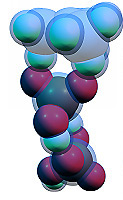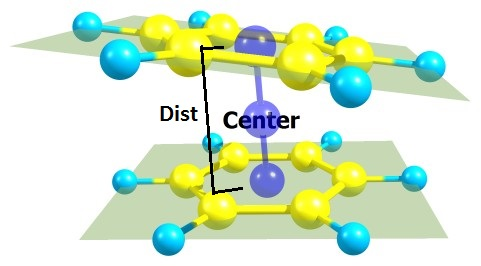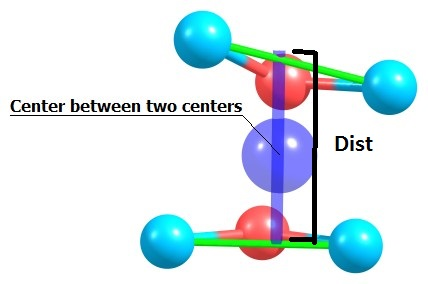## Geometrical parameters calculator (planes and vectors)

Chemcraft can build a plane or a vector comprising selected atoms, and calculate the geometrical parameters with these planes and vectors.

The exact distance between two planes is calculated, when these planes are strictly parallel. The approx distance is calculated as the length of a line between two planes perpendicular to the first plane and passing through the center between the centers of both planes:Similarly, the exact distance between two vectors is the length of a line between these vectors perpendicular to the first vector and passing through the center between the centers of both vectors:BACK